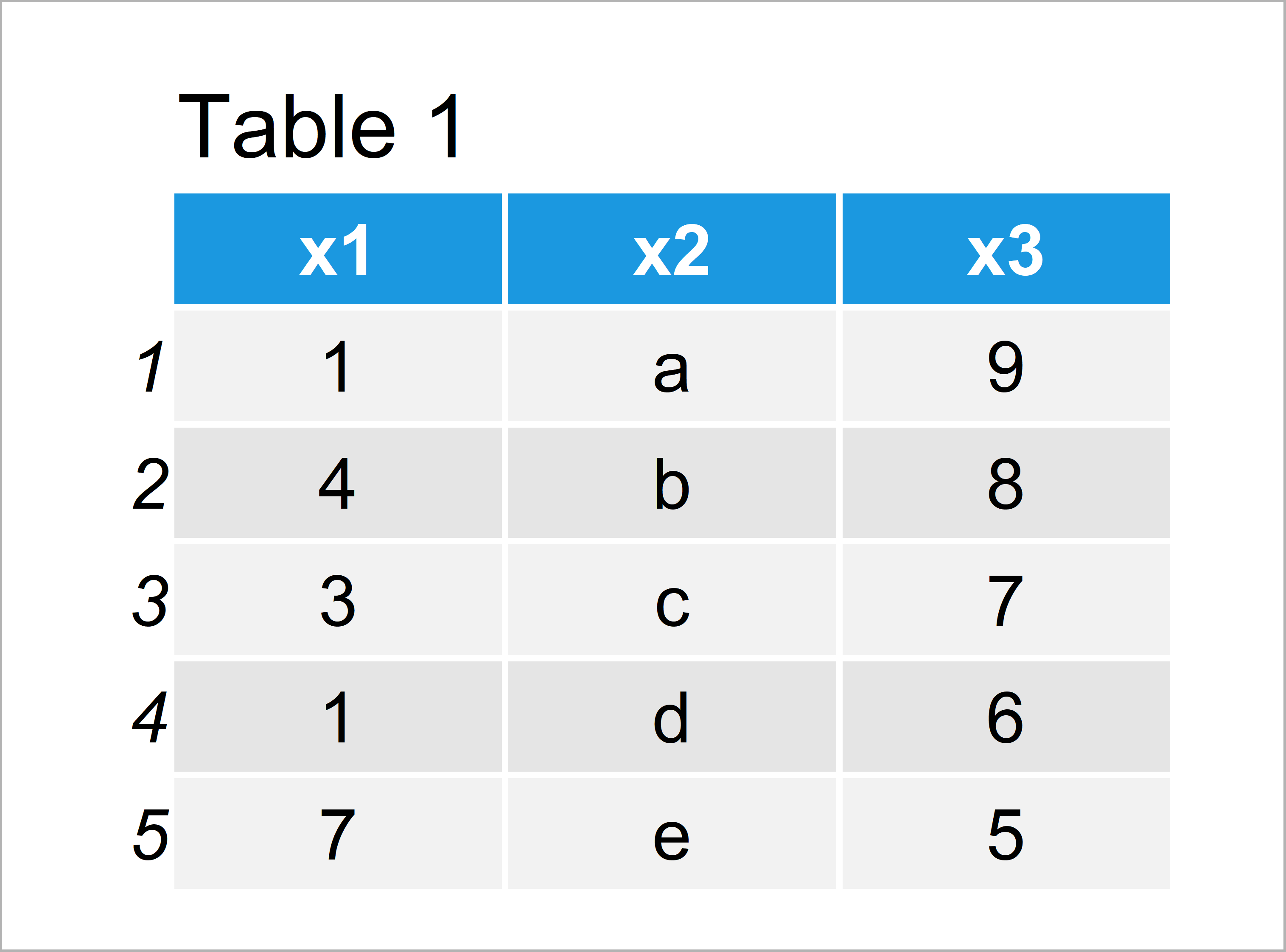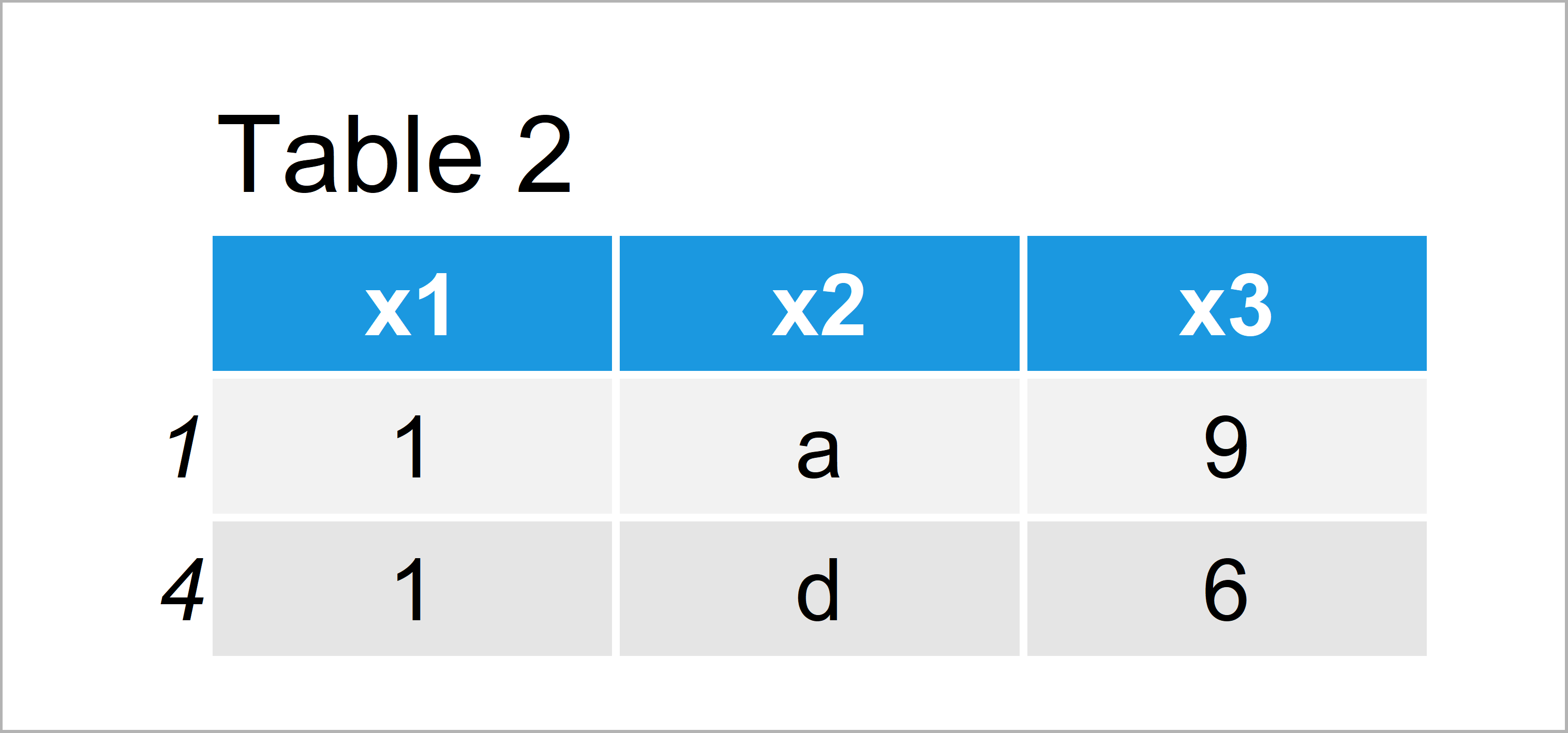# Return Data Frame Row Based On Value in Column in R (Example)

It’s time to dive into the example!

## Creating Example Data

The following data will be used as basement for this R programming tutorial:

```data <- data.frame(x1 = c(1, 4, 3, 1, 7),    # Create example data
x2 = letters[1:5],
x3 = 9:5)
data                                         # Print example data```As you can see based on Table 1, our exemplifying data is a data frame consisting of five rows and three columns.

## Example: Select Data Frame Rows According to Variable

The following R code illustrates how to create a subset of our data frame based on a specific data frame column.

In this example, we’ll select only rows where the variable x1 is equal to 1:

```data_new <- data[data\$x1 == 1, ]             # Extract certain rows
data_new                                     # Print updated data```Table 2 shows the output of the previous R programming code: A data frame subset where x1 is equal to 1.

## Video & Further Resources

Please accept YouTube cookies to play this video. By accepting you will be accessing content from YouTube, a service provided by an external third party.If you accept this notice, your choice will be saved and the page will refresh.

Summary: You have learned in this post how to extract specific rows based on a variable of a data frame in the R programming language. In case you have further questions, please tell me about it in the comments.

Subscribe to the Statistics Globe Newsletter